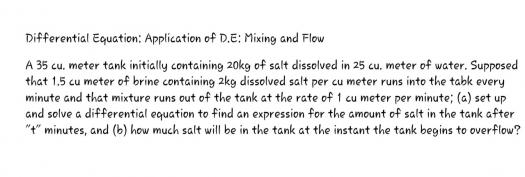# Differential Equation: Application of D.E: Mixing and Flow

1 post / 0 new
Ednalyn DG CarpioDifferential Equation: Application of D.E: Mixing and Flow• Mathematics inside the configured delimiters is rendered by MathJax. The default math delimiters are $$...$$ and $...$ for displayed mathematics, and $...$ and $...$ for in-line mathematics.